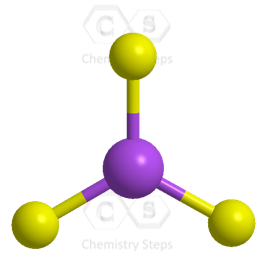## Examples

There are 4 + 3×6 + 2 = 24 electrons. The carbon goes in the middle, and the oxygens take 6 electrons each as three lone pairs:The carbon lacks an octet, so we use a lone pair from one oxygen to make a double with it. The other two oxygen are then negatively charged:The central atom has 3 atoms and no lone pairs, therefore, both geometries are trigonal planar.The steric number (the sum of the number of the atoms and lone pairs) of the carbon is 3 which corresponds to sp2-hybridization.

1675351093